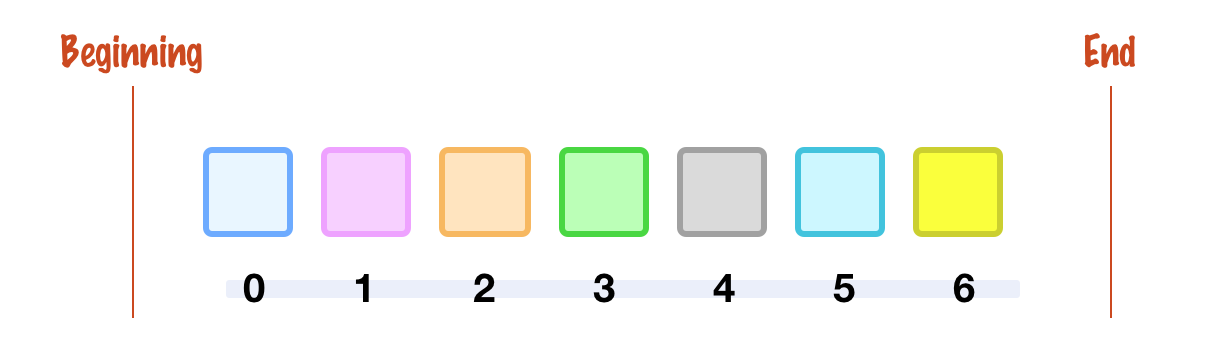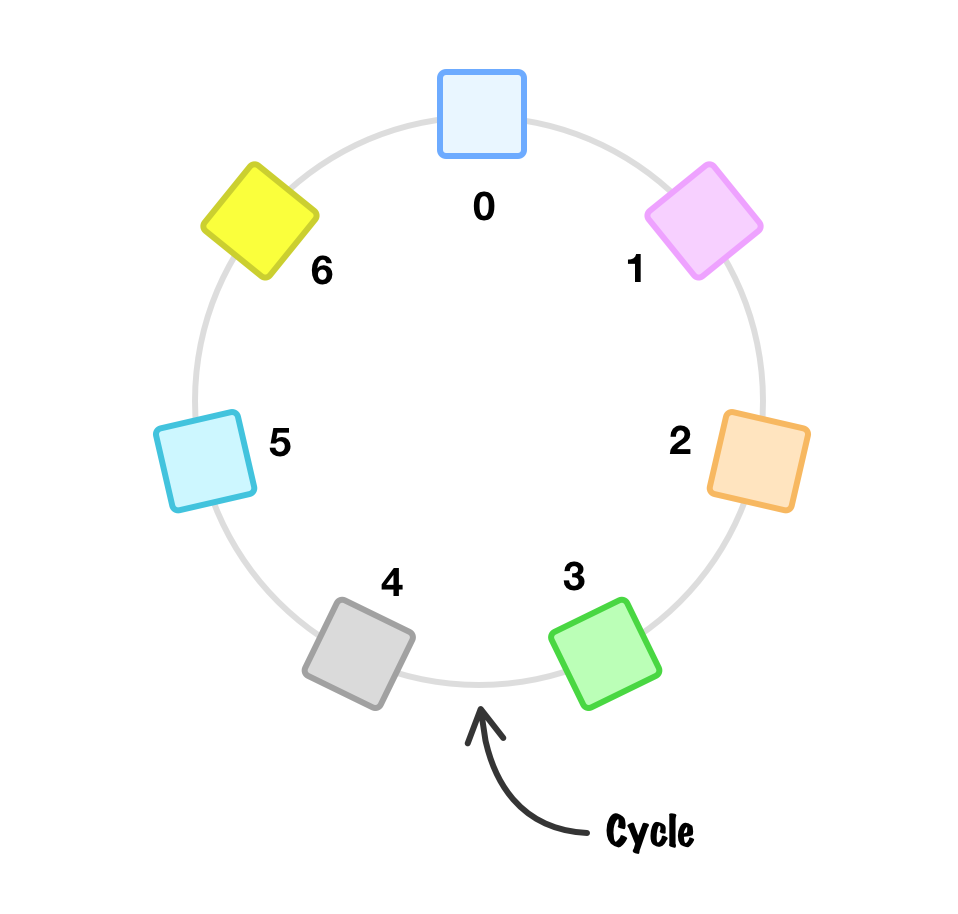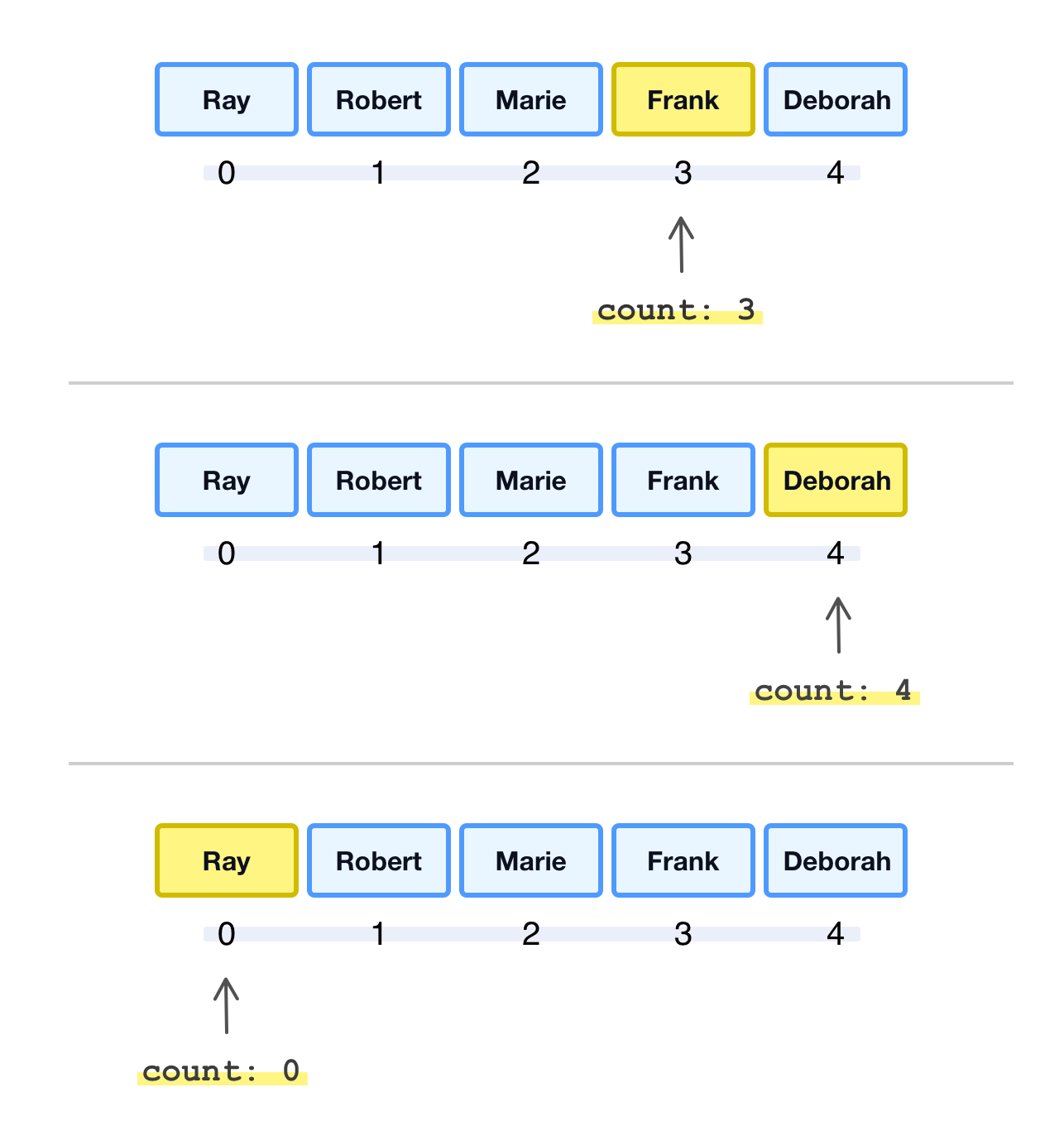﻿ Infinitely Cycle Through an Array

# Infinitely Cycle Through an Array

by kirupa   |    filed under Arrays

When we think of our arrays, we think of them having a finite beginning and end. In the looping through an array article, we looked at the various looping mechanisms we have for going through each item in our array. In all of these mechanisms, we started at the beginning and stopped at the end. For many cases, thinking about our arrays as being finite with a beginning and end is limiting:Instead, what if we think of our arrays as being a cycle where the end and the beginning are more fluid:Now, why might we ever want to do this? There are many cases where treating our arrays as a cycle is appropriate. Imagine having an array of colors that you want to repeatedly animate through. Or, there is a finite list of items in the DOM whose values you want to continuously update. What if you are building a carousel component whose items will infinitely loop? I am sure you can probably come up with more cases, but the important detail is that extending our view of arrays to be a cycle will come in handy. Let's spend the next few sections learning how to work with our arrays in a cyclical world.

Onwards!

## Array Cycling

To make all of this array cycling business easier to wrap our brains around, let's start with an example:

``````<!DOCTYPE html>
<html lang="en">

<meta charset="UTF-8">
<meta name="viewport" content="width=device-width, initial-scale=1.0">
<title>Cycling Array</title>

<body>
<div>

</div>

<script>
let names = ["Ray", "Robert", "Marie", "Frank", "Deborah"];

function cycleArray() {
console.log("Hello!");
}

setInterval(cycleArray, 200);
</script>
</body>

</html>``````

If you create a new HTML document and test this example out by pasting all of the above code and markup into it, you'll see the Console continuously printing the word Hello! to the screen:Take a moment to look at the code. What makes this example work is the setInterval timer function that calls the cycleArray function every 200 milliseconds. It is this cycleArray function that currently prints Hello! to the Console, and it is here we are going to modify our code to continuously print a value from an array instead.

### Counter-Based Approach

One approach to cycling through our array is to have a counter that keeps track of where in the array we are, essentially mimicking the index position. The following is our names array from our example visualized with these details called out:When we reach the end of the array, the counter resets and we start running through the arrray from the beginning and start the cycle all over again:Modifying our script from earlier, the following is what the counter-based array cycling approach looks like:

``````let names = ["Ray", "Robert", "Marie", "Frank", "Deborah"];
let count = 0;

function cycleArray() {
let name = names[count];
console.log(name);

// increment our counter
count++;

// reset counter if we reach end of array
if (count === names.length) {
count = 0;
}
}

setInterval(cycleArray, 200);``````

Each time cycleArray gets called, we use the current value of the count variable to access the item from our array:

``````function cycleArray() {
let name = names[count];
console.log(name);

// increment our counter
count++;

// reset counter if we reach end of array
if (count === names.length) {
count = 0;
}
}``````

We then increment the count variable so that the next item from our array will get accessed the next time cycleArray is called:

``````function cycleArray() {
let name = names[count];
console.log(name);

// increment our counter
count++;

// reset counter if we reach end of array
if (count === names.length) {
count = 0;
}
}``````

When the value of count equals the total number of items in the array, it is time to start the counting all over by setting count back to 0:

``````function cycleArray() {
let name = names[count];
console.log(name);

// increment our counter
count++;

// reset counter if we reach end of array
if (count === names.length) {
count = 0;
}
}``````

This counter based approach will cycle through the contents of our array forever if we never stop calling cycleArray.

### Remainder Approach

The counter approach we saw earlier works, but there is an approach that is far simpler and more flexible that we can use. This is an approach that uses the remainder (aka %) operator. How does this help with our array cycling goal? Let's see for ourselves by replacing the current code inside our script tag with the following:

``````let myArray = ["a", "b", "c", "d", "e", "f", "g", "h", "i", "j"];
let count = 0;

function cycleArray() {
let index = count % myArray.length;
console.log(myArray[index]);

count++;
}

setInterval(cycleArray, 200);``````

If we run this example in the browser, what we'll see in our console are all the letters from myArray looping through. The key lines responsible for this working are the following:

``````let myArray = ["a", "b", "c", "d", "e", "f", "g", "h", "i", "j"];
let count = 0;

function cycleArray() {
let index = count % myArray.length;
console.log(myArray[index]);

count++;
}

setInterval(cycleArray, 200);``````

Each time cycleArray gets called, we calculate the current index position with count % myArray.length. By relying on the remainder of what happens when the current count value is divided by our array length using myArray.length, what we get is an index position that always stays within the bounds of our array. That's the nice thing about remainders. It doesn't matter how large the value of count gets. The remainder will always be a value between 0 and myArray.length - 1, which coincidentally is also the index number range for an array. By relying on this approach, we now have an open door for other array activities that are now much simpler.

If we define a function to access our array items, we no longer have to worry about clamping the input to stay within our array's bounds. Take a look at the following readItem function:

``````let myArray = ["a", "b", "c", "d", "e", "f", "g", "h", "i", "j"];

let arrayItem = this[index % this.length];

return arrayItem;
}

The readItem function takes an index value as the argument, and this index position can be any arbitrarily large positive number. What gets returned is still a value from our array based on what the remainder calculation returns for a valid index position. When working with large datasets where a subset of the data may be repeated, this generic approach for accessing an array item will come in very handy.

When iterating through our array, we commonly start at the beginning with the first item and move our way around. When we are in a world where our arrays don't have a fixed starting or ending point, we have a lot of flexibility to start our array cycling from any item inside the array. If we look at our earlier cycleArray function, we can modify it with an arbitrary starting point defined by the start variable:

``````let myArray = ["a", "b", "c", "d", "e", "f", "g", "h", "i", "j"];
let count = 0;
let start = 3; // start from the 4th item

function cycleArray() {
let index = (start + count) % myArray.length;
console.log(myArray[index]);

count++;
}

setInterval(cycleArray, 200);``````

Our start variable is set to 3, and this means the first item we will start cycling from will be the 4th one, the letter e. From here on out, the cycling will just continue as described earlier. The value of count will keep increasing, and the remainder-based approach will ensure we always stay within the bounds of our array.

### Cycling Through a Subset of an Array

The last bit we will focus on is how to cycle through just a part of our array. Let's keep our same myArray as before, but what we want to do is cycle only through the letters f through h:There are several ways to think about how to solve this. My favorite way is to think about the letters f, g, and h as being the array we cycle through and ignore the larger myArray altogether. We can segment our larger array to only focus on these three items using the starting point and number of items to cycle through as parts of our implementation. Take a look at the following snippet with the key lines highlighted:

``````let myArray = ["a", "b", "c", "d", "e", "f", "g", "h", "i", "j"];

let count = 0;
let start = 5;
let itemsToGoThrough = 3;

function cycleSubArray() {
let index = (start + count) % myArray.length;
console.log(myArray[index]);

count++;

if (count == itemsToGoThrough) {
count = 0;
}
}

setInterval(cycleSubArray, 200);``````

Our start variable points to the letter f, and we track the count variable to see how many letters we need to cycle through. For cycling through f, g, and h, the number of items is 3, and that is what the itemsToGoThrough variable stores. In the body of our cycleSubArray, we check if the value of count has reached the value of itemsToGoThrough. If count has reached that value, we reset the count variable back to 0 to have it start at the letter f again.

## Conclusion

When learning about arrays, thinking about them as having a strict beginning and end is a good first step, but it also adds some overhead that will start getting in the way in many situations. That is why thinking about arrays as a cycle is a game changer. Well, not quite a game changer in the literal sense, but it does make many types of tasks involving arrays and repetitive tasks much easier.

Got a question or just want to chat? Comment below or drop by our forums (they are actually the same thing!) where a bunch of the friendliest people you'll ever run into will be happy to help you out!